# Outliers-Part 3:Outliers in Regression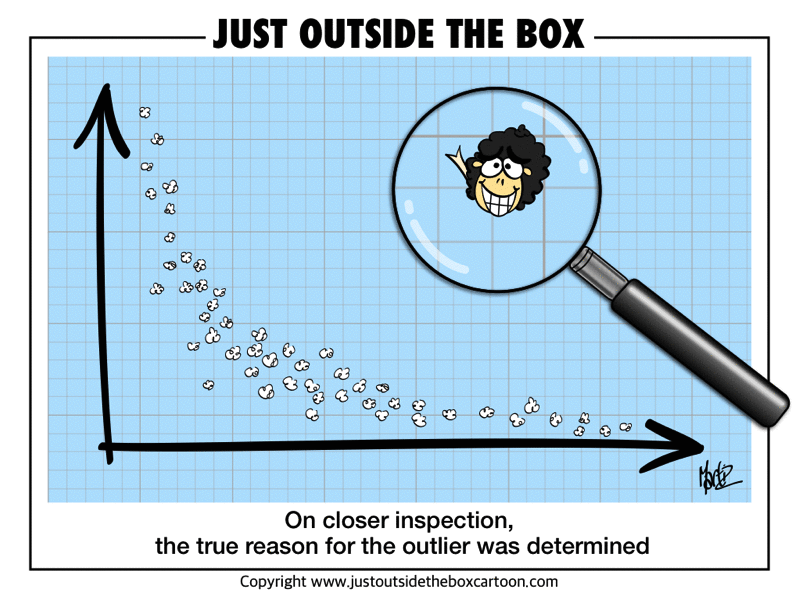Figure 0.1: Outliers

In the previous blog post, we’ve discussed the philosophy of outliers part 1 and outlier detection univariate methods part 2. In this 3rd post, we are going to discuss more technical details of the outlier detection in regression.

A observation that is substantially different from all other ones can make a large difference in the results of regression analysis.

Outliers play important role in regression. More importantly, separated points can have a strong influence on statistical models-deleting outliers from a regression model can sometimes give completely different results.

Let’s see the example below. This example uses the dataset-cars.

# original data
cars1 <- cars[1:30, ]

# introduce outliers.
cars_outliers <- data.frame(speed=c(19,19,20,20,20),
dist=c(190, 186, 210, 220, 218))

cars2 <- rbind(cars1, cars_outliers)  # data with outliers.

# Plot of data with outliers.
par(mfrow=c(1, 2))

plot(cars2$speed, cars2$dist,
xlim=c(0, 28), ylim=c(0, 230),
main="With Outliers",
xlab="speed", ylab="dist",
pch="*", col="red", cex=2)

# regression reference line
abline(lm(dist ~ speed, data=cars2), col="blue", lwd=3, lty=2)

# Plot of original data without outliers.
# Note the change in slope (angle) of best fit line.
plot(cars1$speed, cars1$dist,
xlim=c(0, 28), ylim=c(0, 230),
main="Outliers removed \n A much better fit!",
xlab="speed", ylab="dist",
pch="*", col="red", cex=2)

abline(lm(dist ~ speed, data=cars1), col="blue", lwd=3, lty=2)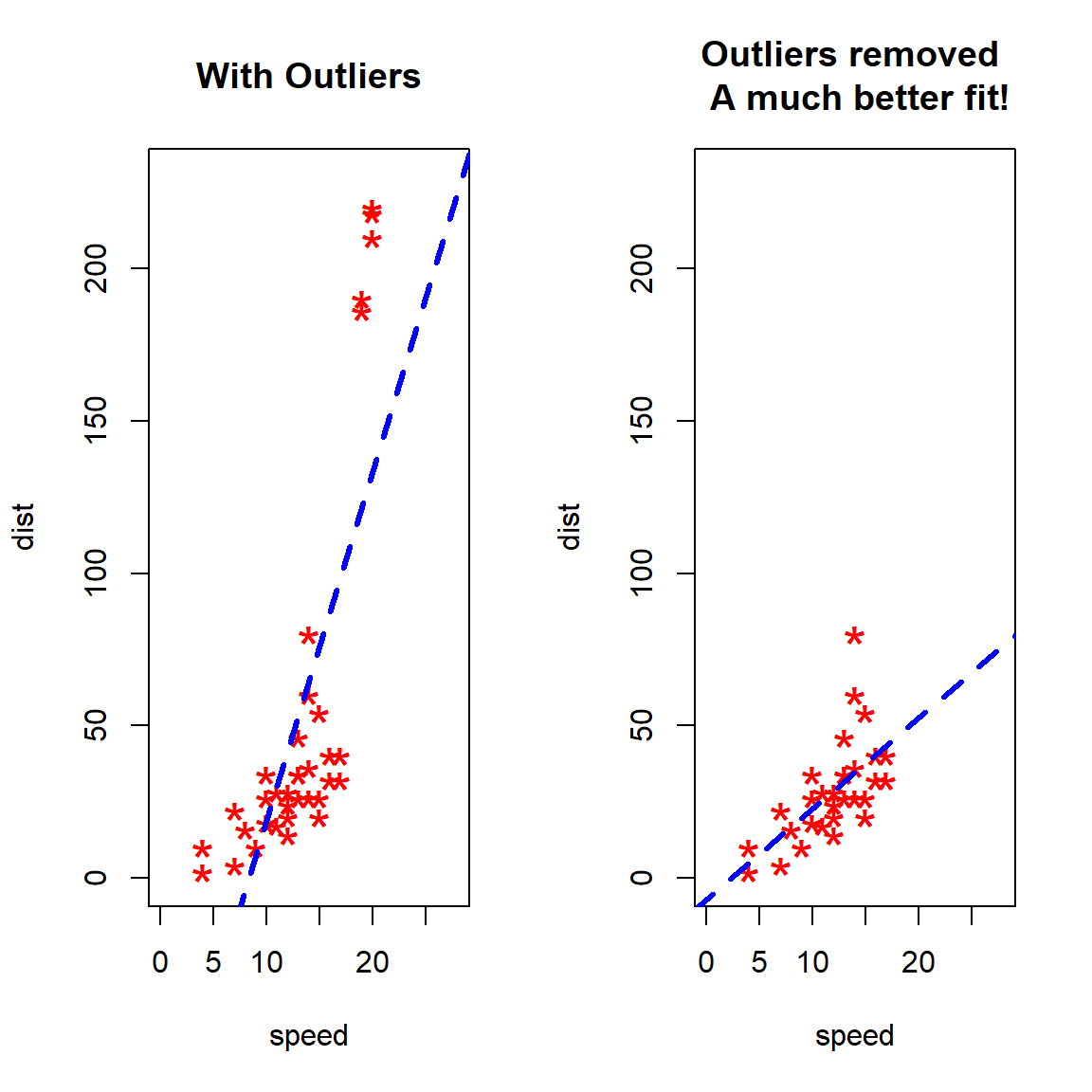Despite all this, as much as you’d like to, it is NOT acceptable to drop an observation just because it is an outlier. They can be legitimate observations and are sometimes the most interesting ones. Like what I stated in the previous posts, it’s important to investigate the nature of the outlier before deciding. Once the outliers or unusual observations are detected, the best way to start is to ask whether the outliers even make sense, especially given the other variables you’ve collected.

# 1 Types of Unusual Observations

## 1.1 Regression Outliers

It is common practice to distinguish between two types of outliers. Outliers in the response variable (DV) represent model failure. Such observations are called outliers. A regression outlier is an observation that has an unusual value of the dependent variable $$Y$$, conditional on its value of the independent variable $$X$$. A regression outlier will have a large residual but not necessarily affect the regression slope coefficient.

See the Figure (a) below. This is an example of an outliers without influence.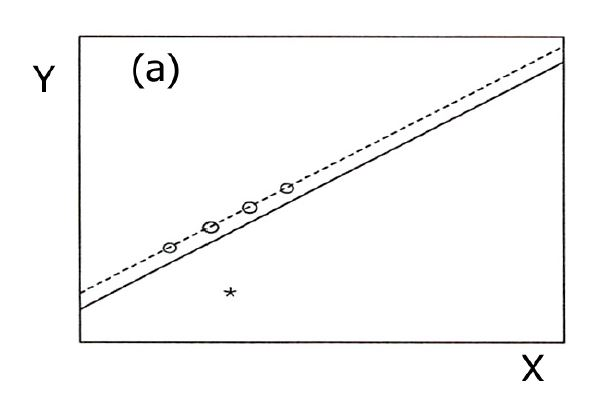Figure 1.1: Figure (a): Outlier without influence

Although its Y value is unusual given its X value, it has little influence on the regression line because it is in the middle of the X-range.

## 1.2 Leverage

Outliers with respect to the predictors (IV) are called leverage points. An observation that has an unusual $$X$$ value-i.e., it is far from the mean of $$X$$ -has leverage on (i.e., the potential to influence) the regression line. The further away from from the mean of $$X$$ ($$\bar{x}$$, either in a positive or negative direction), the more leverage an observation has on the regression fit. High leverage does not necessarily mean that it influences the regression coefficients.

It is possible to have a high leverage and yet follow straight in line with the pattern of the rest of the data. High leverage observations can affect the regression model, too. Their response variables need not be outliers.

See the Figure (b) below. This is an example of high leverage observation.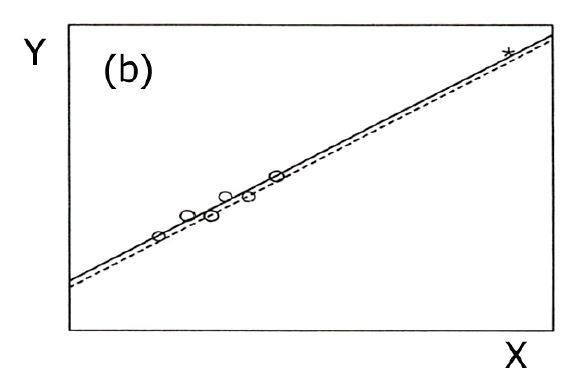Figure 1.2: Figure (b): High leverage

because it has a high value of X. However, because its value of Y puts it in line with the general pattern of the data it has no influence.

## 1.3 Influential Observations

High leverage points that actually influence the slope of the regression line are called influential points. Only when an observation has high leverage and is an outlier in terms of Y-value will it strongly influence the regression line. In other words, it must have an unusual $$X-$$value with an unusual $$Y-$$value given its $$X-$$value. In such cases both the intercept and slope are affected, as the line chases the observation.

$Influence = Leverage \times Discrepancy$ See the Figure (c) below. This is an example of a combination of discrepancy (unusual Y value) and high leverage (unusual X value) observation.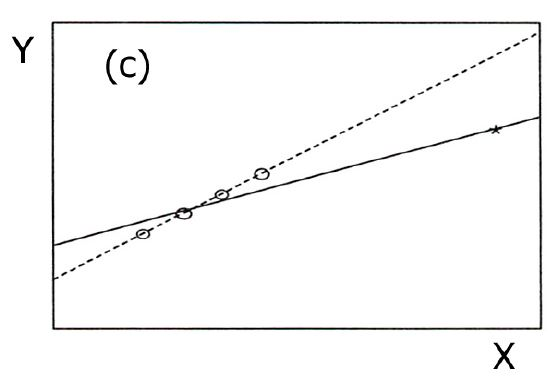Figure 1.3: Figure (c): Combination of discrepancy (unusual Y value) and leverage (unusual X value)

This observation results in strong influence. When this case is deleted both the slope and intercept change dramatically..

In summary, outliers in regression are:

• Outliers are points that fall away from the cloud of points.
• Outliers that fall horizontally away from the center of the cloud are called leverage points
• High leverage points that actually influence the slope of the regression line are called influential points
• In order to determine if a point is influential, visualize the regression line with and without the point. Does the slope of the line change considerably? If so, then the point is influential. If not, then it’s not.

## 1.4 Good vs. Bad Leverage

In regression it helps to make a distinction between two types of leverage points: good and bad.

A good leverage point is a point that is unusually large or small among the X values but is not a regression outlier. That is, the point is relatively removed from the bulk of the observation but reasonably close to the line around which most of the points are centered. A good leverage point has limited effect on giving a distorted view of how majority of points are associated. Good leverage points improve the precision of the regression coefficients.

A bad leverage point is a point situated **far from the regression line* around which the bulk of the points are centered. Said another way, a bad leverage point is a regression outlier that has an X value that is an outlier among X values as well (it is relatively far removed from the regression line). Bad leverage point has grossly effect estimate of the slope of the regression line if an estimator with a small breakdown point is used. Bad leverage points reduce the precision of the regression coefficients.

Leverage points do not necessarily correspond to outliers.

Observations whose inclusion or exclusion result in substantial changes in the fitted model (coefficients, fitted values) are said to be influential.

We are mostly concerned with regression outliers, that is, cases for which ($$x_{k_{1}},...x_{k_{p}},y_{k}$$) deviates from the linear relation followed by the majority of the data, taking into account both the explanatory variable and the response variable simultaneously. A leverage point is then still defined as a point ($$x_{k_{1}},...x_{k_{p}},y_{k}$$) for which ($$x_{k_{1}},...x_{k_{p}}$$) is outlying with respect to the ($$x_{i_{1}},...x_{i_{p}}$$) in the data set.

# 2 Detecting Influential Observations

Many numerical and graphic diagnostics for detecting outliers and influential cases on the fit have been suggested.

## 2.1 Graphic diagnostics

In the simple regression model, one can make a plot of the $$(x_{i},y_{i})$$, which is called a scatterplot, in order to visualize the data structure. Many people will argue that regression outliers can be discovered by looking at the least squares residuals. Unfortunately, this is not true when the outliers are leverage points. If one would apply a rule like “delete the points with largest LS residuals”, then the “good” points would have to be deleted first. Often, influential points remain hidden, because they do not always show up in the usual LS residual plot.

### 2.1.1 A scatter plot with Confidence Ellipse

A scatter plot of $$x$$ versus $$y$$ is used to visualize the conditional distribution $$y|x$$. For the simple linear regression model, by far the most effective technique for checking the assumption of the model is to make a scatterplot of $$x$$ versus $$Y$$ and residual plot of $$x$$ versus $$r_{i}$$.

Departure from linearity in the suggests the simple linear regression model is not adequate. Points in the residual plot should scatter about the line $$r=0$$ with the pattern. If curvature is present or if the distribution of the residuals depends on the value of x, then the simple linear model is not adequate. Usually, a confidence ellipse is drawn around the point cluster center coordinates. The rule of thumb is $$0.95$$. Points outside of, say, 95% confidence ellipse is labeled as outlier or unusual observations.

data(Davis)
attach(Davis)

model1 <- lm(weight ~ height)

# draw 95% CI ellipse
# from library(car)
# confidenceEllipse(weakliem.model1, levels=0.95,Scheffe=TRUE)
car::dataEllipse(height, weight, levels=0.95, lty=1, col=1,
main = "Height vs. Weight",
xlab="Height", ylab="Weight")

abline(model1, lwd=2, lty=1, col=2)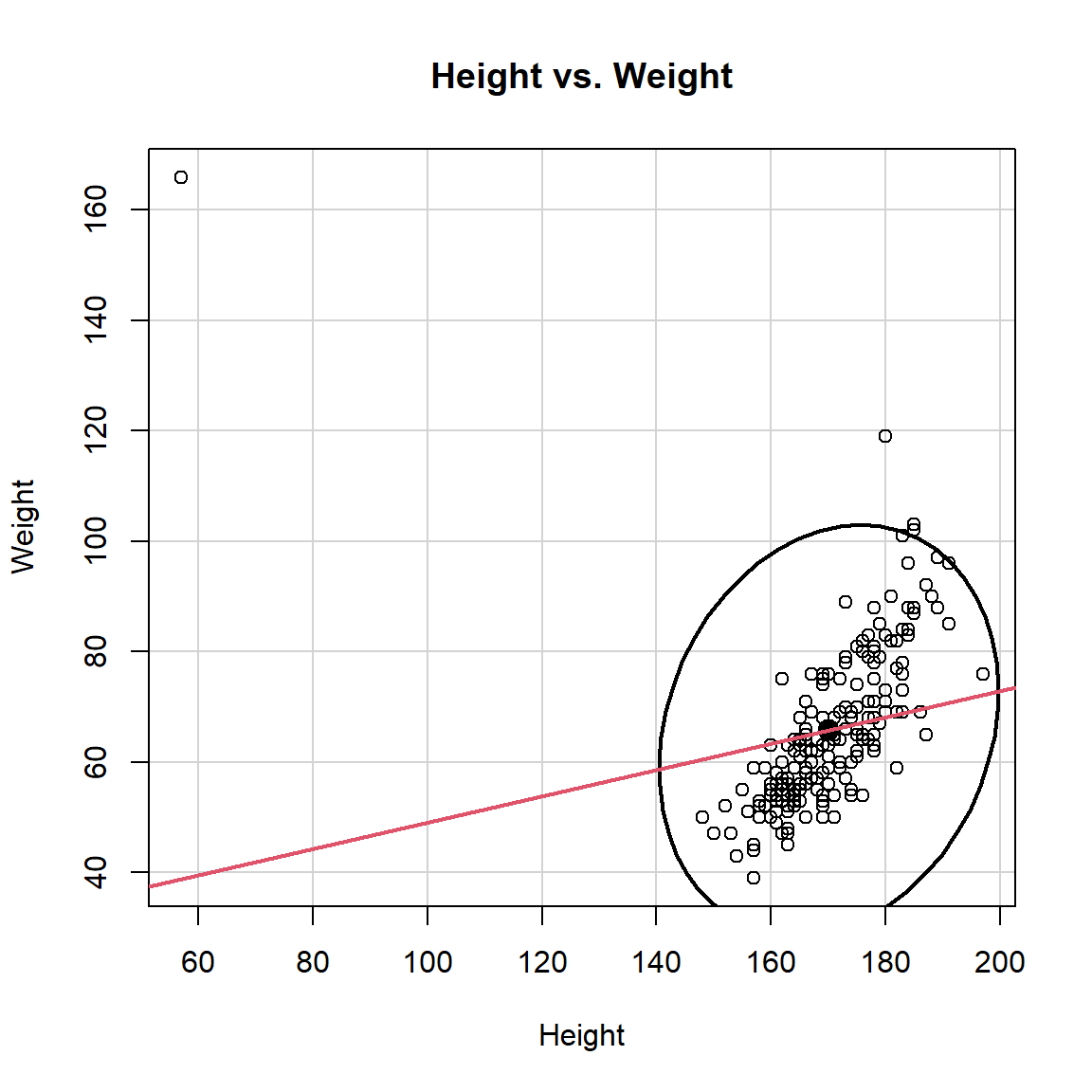### 2.1.2 Quantile Comparison Plots (QQ-Plot)

First, we could use quantile comparison plots (QQ-Plot) to compare the distribution of a single variable to the t-distribution, assessing whether the distribution of the variable showed a departure from normality. Similarly, we can compare the distribution of the studentized residuals from our regression model to the t-distribution.

#### 2.1.2.1 Rule of Thumb

Observations that stray outside of the 95% confidence envelope are statistically significant outliers.

For illustration, R sample data (state.x77) is used in the plot example. This data set give us information such as population size, average income, illiteracy rate, temperature, and murder rate for 50 states in USA.

The qqPlot function from {car} is used to detect outliers. It draws theoretical quantile-comparison plots for variables and for studentized residuals from a linear model. A comparison line is drawn on the plot either through the quartiles of the two distributions, or by robust regression.

library(car)

states <- as.data.frame(state.x77[,c("Murder", "Population",
"Illiteracy", "Income", "Frost")])

# fit the data into the linear regression model
fit1 <- lm(Murder ~ Population + Illiteracy + Income + Frost, data=states)
# summary(fit)

# From our regression model example, we can start
# investigating outliers observation by using Q-Q plot.
car::qqPlot(fit1,labels=row.names(states), id.method="identify",
simulate=TRUE, main="Q-Q Plot")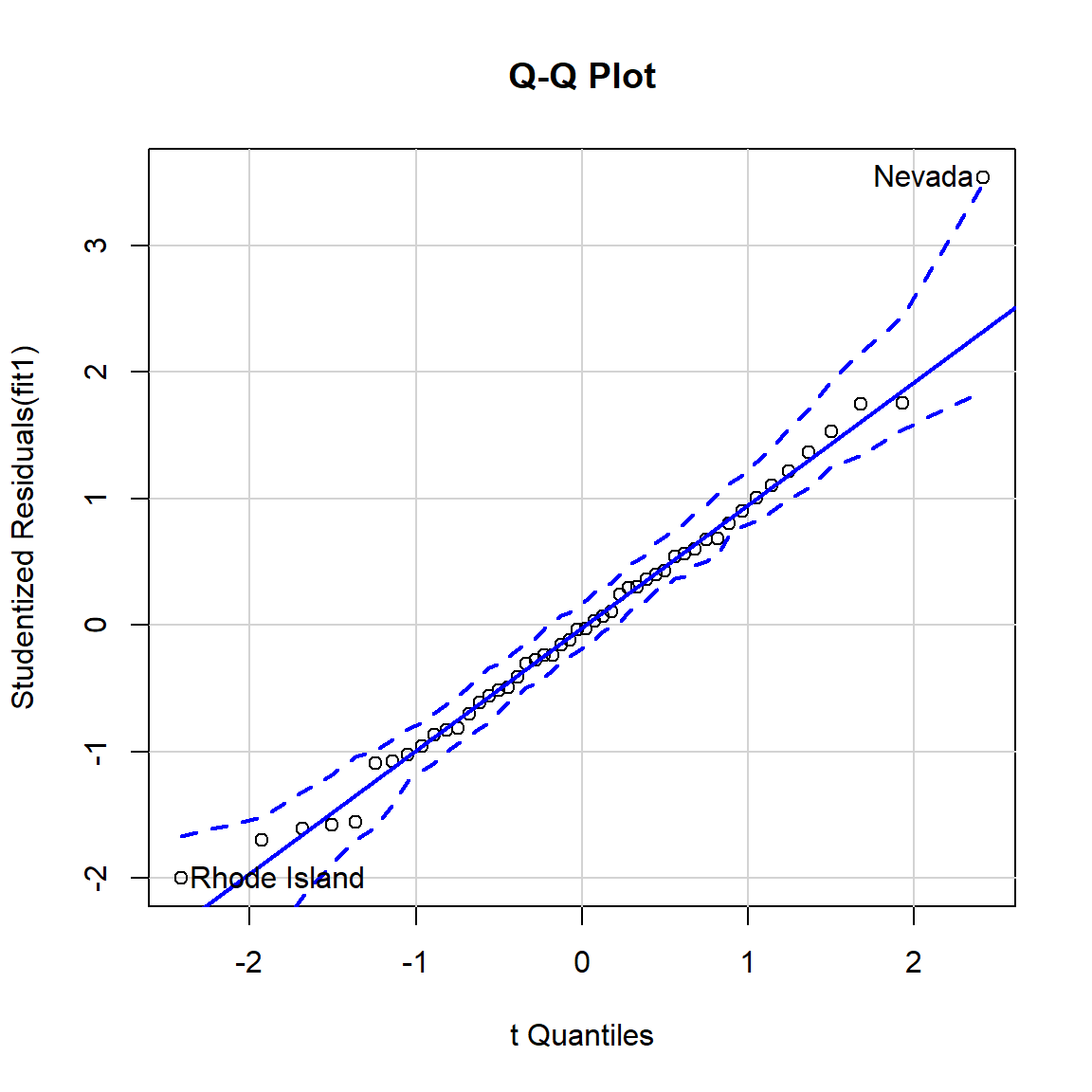##       Nevada Rhode Island
##           28           39

As you can observed the plot above, Nevada (28th observation) and Rhode Island (39th observation) are states that detected as potential outliers.

Subsets of cases can jointly influence a regression line, or can offset each other’s influence. Cook’s D can help us determine joint influence if there are relatively few influential cases.

However, Cook’s D may not be impractical if there are potentially a large number of subsets to explore. Thus, Added-variable plots (also called partial regression plots) provide a more useful method of assessing joint influence.

In applied statistics, a partial regression plot aka Added-variable plots attempts to show the effect of adding another variable to a model that already has one or more independent variables.

When performing a linear regression with a single independent variable, a scatter plot of the response variable against the independent variable provides a good indication of the nature of the relationship. If there is more than one independent variable, things become more complicated. Although it can still be useful to generate scatter plots of the response variable against each of the independent variables, this does not take into account the effect of the other independent variables in the model.

Added-variable plot can be easily created using the avPlots() function from {car} package.

Similar to QQ-Plot example, R sample data (state.x77) is also used in the added-variable plot example.

states <- as.data.frame(state.x77[,c("Murder", "Population",
"Illiteracy", "Income", "Frost")])

# fit the data into the linear regression model
fit1 <- lm(Murder ~ Population + Illiteracy + Income + Frost, data=states)
# It can be easily created using the avPlots() function in car package.
# From the graph below, the straight line in each plot is the actual
# regression coefficient for that predictor variable.
car::avPlots(fit1, ask=FALSE, id.method="identify")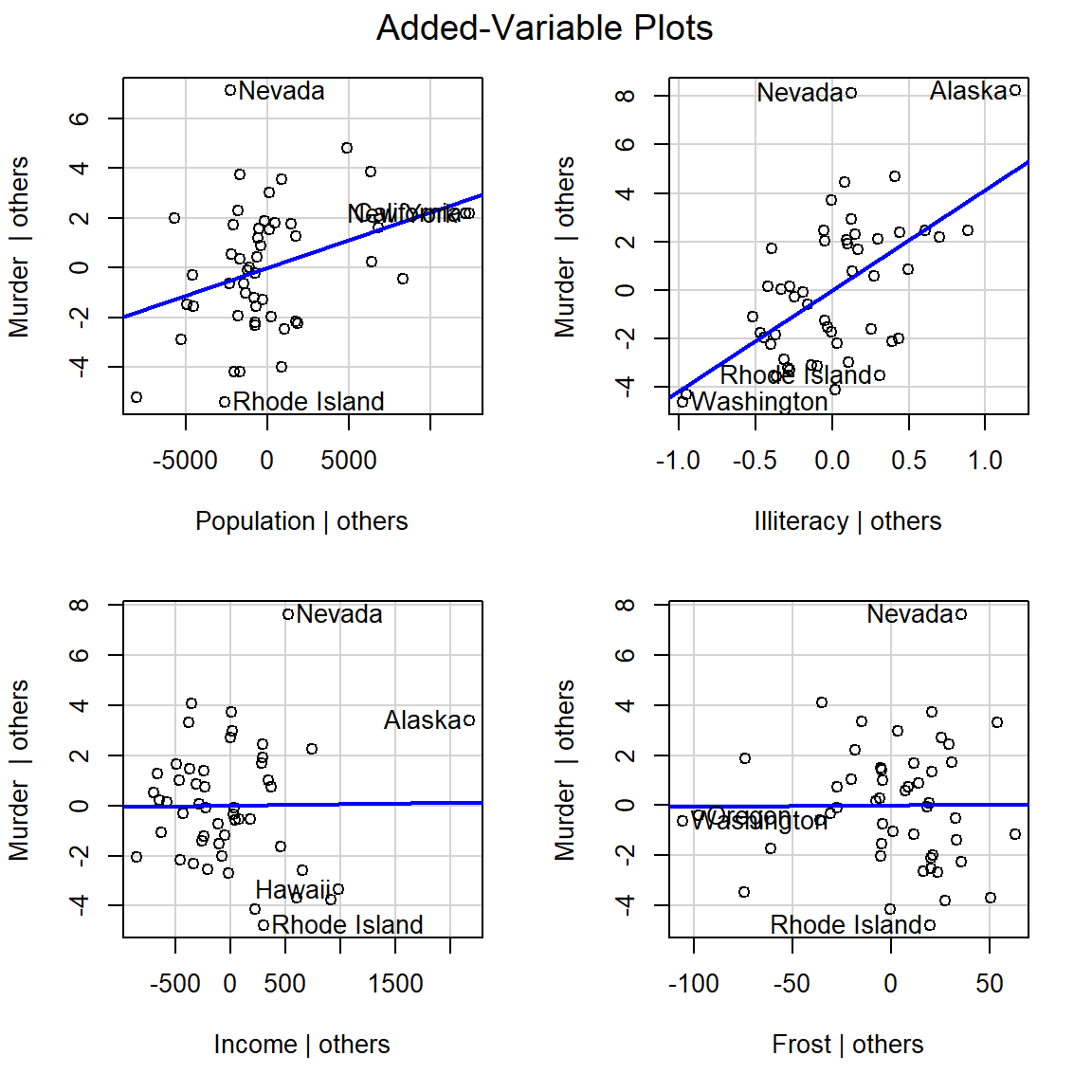We see here that cases-Nevata and Alaska have unusually high Y values given their X’s. Because they are on the extreme of the X-range as well, they are most likely influencing all slopes.

## 2.2 Numerical diagnostics

Diagnostics are certain quantities computed from the data with the purpose of pinpointing influential points, after which these outliers can be removed or corrected. When there are only one a single outlier, some of these methods work quite well by looking at the effect of deleting one point at a time.

### 2.2.1 Hat Matrix

In classical linear regression, the diagonal elements $$h_{ii}$$ of the hat matrix $\mathbf H = \mathbf X(\mathbf X^{T}\mathbf X)^{-1}\mathbf X^{T}$ are used to identify leverage points. The i-th leverage $$h_{i} = H_{ii}$$ is the i-th diagonal element of the hat matrix $$\mathbf H$$.

Rousseeuw and Van Zomeren (1990) report the following monotone relationship between the $$h_{ii}$$ and $$MD_{i}$$

$h_{ii}=[((MD_{i})^2/(n-1))]+[1/n]$ where $$n$$ is the number of observations.

Multiple outliers do not necessarily have large $$MD_{i}$$ values because of the masking effect.

#### 2.2.1.1 Rule of Thumb

Rousseeuw and Leroy (1987) suggest using $$h_{i}>2p/n$$ as benchmarks for leverages.

Some researchers believe Hat-values exceeding about twice the average hat-value should be considered noteworthy.

Hat-value can be easily calculated using the hatvalues() function from {stats} package.

Similar to QQ-Plot example, R sample data (state.x77) is also used in the hat-value example.

# data states
states <- as.data.frame(state.x77[,c("Murder", "Population",
"Illiteracy", "Income", "Frost")])

# fit the data into the linear regression model
fit1 <- lm(Murder ~ Population + Illiteracy + Income + Frost, data=states)

# fit1 <- lm(Murder ~ Population + Illiteracy + Income + Frost, data=states)
# You can compute the high leverage observation by looking at the
# ratio of number of parameters estimated in model and sample size.
# If an observation has a ratio greater than 2-3 times the average ratio,
# then the observation considers as high-leverage points.
# high leverage function

p <- length(coefficients(fit1)) # number of IVs + Intercept
n <- length(fitted(fit1))       # number of observations (N)

# 2-3 times average ratio as cutoff
ratio <- p/n

# display hatvalues
hatvalues(fit1)
##        Alabama         Alaska        Arizona       Arkansas     California
##     0.09819211     0.43247319     0.12264914     0.08722029     0.34087628
##       Colorado    Connecticut       Delaware        Florida        Georgia
##     0.05469964     0.09474658     0.05859637     0.12940379     0.05803142
##         Hawaii          Idaho       Illinois        Indiana           Iowa
##     0.22443594     0.06905425     0.09683616     0.03871740     0.04627447
##         Kansas       Kentucky      Louisiana          Maine       Maryland
##     0.05245106     0.05131253     0.17022388     0.09966458     0.06942663
##  Massachusetts       Michigan      Minnesota    Mississippi       Missouri
##     0.02687166     0.05740920     0.04638897     0.14748152     0.04199088
##     0.05253317     0.04421328     0.09508977     0.06387335     0.06616617
##     New Mexico       New York North Carolina   North Dakota           Ohio
##     0.15998871     0.23298637     0.05218913     0.10529028     0.08652446
##       Oklahoma         Oregon   Pennsylvania   Rhode Island South Carolina
##     0.05117056     0.19057634     0.11185698     0.04562377     0.10445404
##   South Dakota      Tennessee          Texas           Utah        Vermont
##     0.07553161     0.04580225     0.13392638     0.06970746     0.08788063
##       Virginia     Washington  West Virginia      Wisconsin        Wyoming
##     0.03230467     0.21662901     0.05839687     0.04173326     0.06012359
# High leverage function.
highleverage <- function(fit) {

p <- length(coefficients(fit)) # number of IVs + Intercept
n <- length(fitted(fit))       # number of observations (N)
ratio <- p/n
# leverage ratio
plot(hatvalues(fit), main="Index Plot of Ratio")

# 2-3 times the average ratio as cutoff lines.
abline(h=c(2,3)*ratio, col="red", lty=2)

# identify high leverage points from graph.
graphics::identify(1:n, hatvalues(fit), names(hatvalues(fit)))
}

# run function
# Since the graphics::identify function is included, we will not actually run this code in the rmarkdown.
# highleverage(fit1)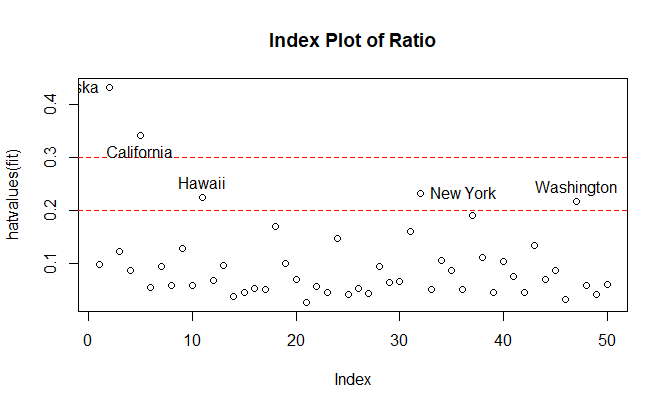Figure 2.1: High leverage plot example

The graph above indicates that Alaska and California have the highest ratio. It means these two states are quite unusual if we compared their predictor values compared to other states. Here is why:

• For Alaska, this state has a much higher income compared to other states, while having smaller population and lower temperature.

• For California, this state has much a bigger population than other states, while having higher income and higher temperature.

Both of these states are indeed quite particular compared to the other 48 states. One thing to remember that not all high-leverage observations are influential observations. It will depend if they are outliers or not.

Unusual observations typically have large residuals but not necessarily so-high leverage observations can have small residuals because they pull the line towards them.

The ordinary or simple residuals (observed - predicted values) are the most commonly used measures for detecting outliers.

### 2.2.2 Standardized Residuals

Standardized Residuals are the residuals divided by the estimates of their standard errors. They have mean 0 and standard deviation 1. Unusual observations typically have large residuals but not necessarily so-high leverage observations can have small residuals because they pull the line towards them:

$V(E_{i})=\sigma_{\epsilon}^2(1-h_{i})$

Standardized residuals provide one possible, though unsatisfactory, way of detecting outliers.

$E_{i}^{'}=\frac{E_{i}}{S_{E}\sqrt{1-h_{i}}}$

#### 2.2.2.1 Rule of Thumb

A large standardized residual ($$d_{i}>3$$) indicates the existence of outliers (Montgomery, D.C., et al.).

However, The numerator and denominator are not independent in the standardized residual. Thus, $$E_{i}^{'}$$ does not follow a t-distribution: If $$|E_{i}|$$ is large, the standard error is also large:

$S_{E}=\sqrt{\sum{E_{i}^2}/(n-k-1)}$

### 2.2.3 Studentized Residuals

If we refit the model deleting the $$i_{th}$$ observation we obtain an estimate of the standard deviation of the residuals $$S_{E(-1)}$$ (standard error of the regression) that is based on the n-1 observations. Then the studentized residuals $$E_{i}^{*'}$$ is calculated. It has an independent numerator and denominator: $E_{i}^{*}=\frac{E_{i}}{S_{E(-i)}\sqrt{1-h_{i}}}$ Studentized residuals follow a t-distribution with $$n−k−2$$ degrees of freedom. This method is suitable when there are several cases that might be outliers.

#### 2.2.3.1 Rule of Thumb

• $$r_{i}>3$$ indicates that $$e_{i}$$ is an outlier, $$i =1,2,…,n$$ (Montgomery, D. C., et al.).

• Also, some researchers suggested that attention should be paid to studentized residuals that exceed +2 or -2 and get even more concerned about residuals that exceed $$|2|$$. Observations that have a studentized residual outside the $$\pm2$$ range are considered statistically significant at the 95% a level and even yet more concerned about residuals that exceed $$|3|$$.

Now lets look at the leverage’s to identify observations that will have potential great influence on regression coefficient estimates. Generally, a point with leverage greater than $$\frac{2k+2}{n}$$ should be carefully examined, where $$k$$ is the number of predictors (IV) and $$n$$ is the number of observations.

#### 2.2.3.2 Studentized Residuals-the Bonferroni adjustment

Since the furthest outlier are going to be selected, it is not legitimate to use a simple t-test. We would expect that $$5%$$ of the studentized residuals would be beyond $$t_{.025}\pm2$$ by chance alone. In order to fix this, we can make a Bonferroni adjustment to the p-value. The Bonferroni p-value for the largest outlier is: $$p=2np$$where $$p$$is the unadjusted p-value from a t-test with n-k-2 degrees of freedom. A special t-table is needed if you do this calculation manually, but this is done for you automatically, using the outlierTest function in the {car} package.

The Bonferroni-adjusted outlier test in {car} tests the largest absolute studentized residual.

states <- as.data.frame(state.x77[,c("Murder", "Population",
"Illiteracy", "Income", "Frost")])

# fit the data into the linear regression model
fit1 <- lm(Murder ~ Population + Illiteracy + Income + Frost, data=states)

# Applying outlierTest function is helping us to
# confirm if potential outliers are indeed outliers.
car::outlierTest(fit1)
##        rstudent unadjusted p-value Bonferroni p
## Nevada 3.542929         0.00095088     0.047544

As you can see the studentized residual and its Bonferroni’s P-value, state-Nevada could be the potential outliers.

In {car} package, there isn’t a single-alone function to plot studentized residual, but through function-influencePlot and influenceIndexPlot, you can incorporate all information from outlier, high-leverage, and influential observations into a single informative plot.

# id.n indicates how many the most unusual observations are identified.
car::influenceIndexPlot(fit1, id.n=3)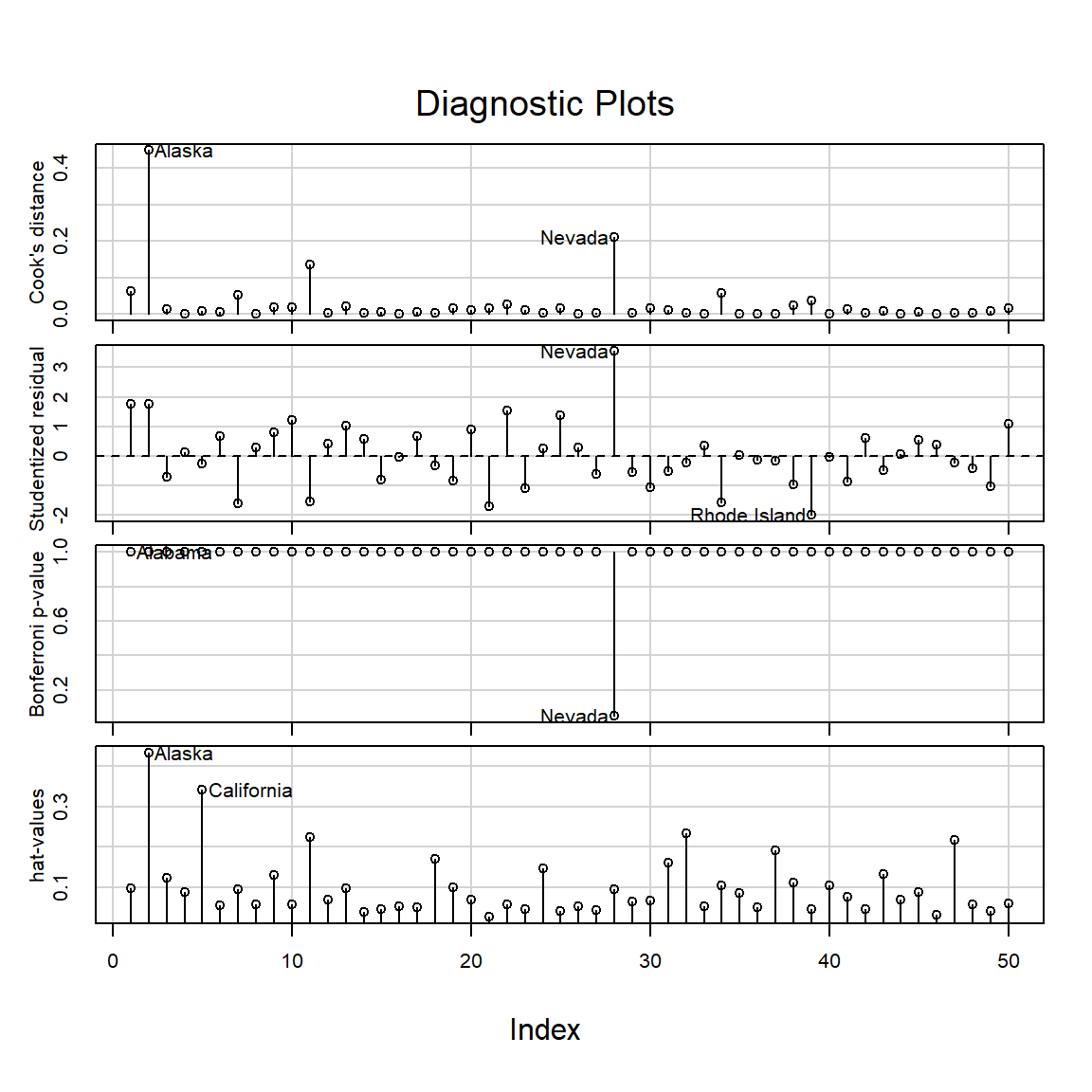In this diagnostic plots, you can find 4 different plots:

• Hat-value
• Bonferroni’s P-value
• Studentdized residual
• Cook’s Distance

The id.n keyword indicates how many the most unusual observations are identified.

### 2.2.4 DFBeta and DFBetas

DFBeta and DFBetas are a direct measure of the influence of an observation on regression parameter estimates. Remember that an influential observation is one that combines discrepancy with leverage. so, DFBeta examines how regression coefficients change if outliers are omitted from the model. $$D_{ij}$$ is often termed $DFBeta_{ij}$ as: $D_{ij} = b_{j} - b_{j(-1)}$ where $$b_{j}$$ are for all the data and the $$b_{j(-1)}$$ are with the $$i_{th}$$ observation removed. These differences are usually scaled by (omitted) estimates of the standard error of $$b_{j}$$:

$d_{ij}^{*}=\frac{d_{ij}}{s_{-i}(b_{j})}$ The $$d_{ij}$$ are often termed DFBETA and the $$d_{ij}^{*}$$ are called DFBETAS.

#### 2.2.4.1 Rule of thumb

A standard cut-off for DFBetas is: $$D_{ij}^{*} = 2\times n^{-.5}$$.

The R function dfbetas from {stats} package is used to calculate DFBetas value.

# data states
states <- as.data.frame(state.x77[,c("Murder", "Population",
"Illiteracy", "Income", "Frost")])

# fit the data into the linear regression model
fit1 <- lm(Murder ~ Population + Illiteracy + Income + Frost, data=states)

## an influential observation is one that
#  combines discrepancy with leverage.
fit1.dfbetas <- dfbetas(fit1)

# c(2,3) specifies the coefficients of interests (Population and illiteracy)
plot(fit1.dfbetas[,c(2,3)],
xlim = c(-2,2),ylim=c(-1,1),
main="DFBetas for the Population and Illiteracy coefficients")

# adds the rule of thumb cut-off line
abline(h=2/sqrt(length(states)), lty=2)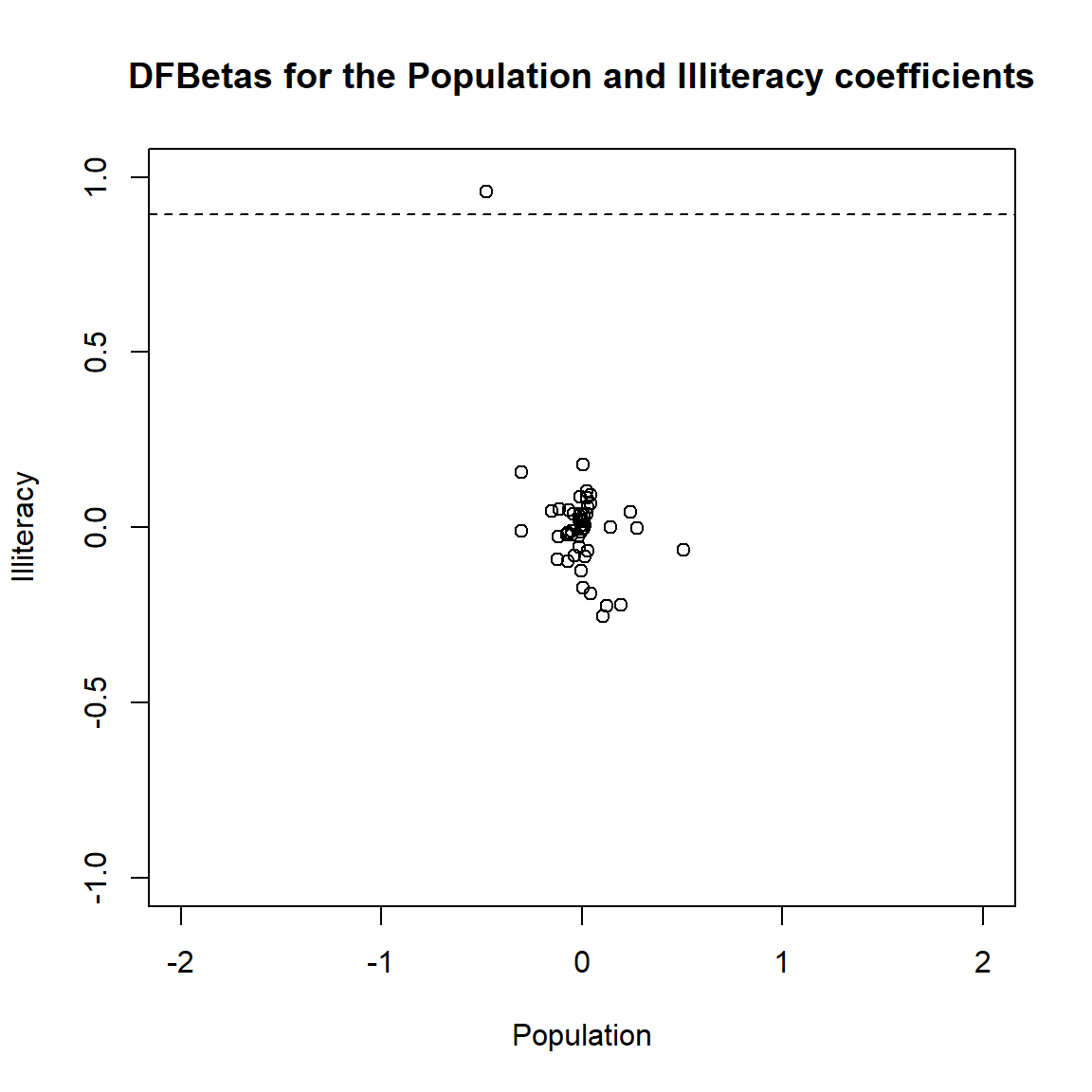Then, you can identify the influential point above the cut-off line.

### 2.2.5 Robust Distance

The robust distance is defined as

$RD(x_{i})=\sqrt{[x_{i} - \mathbf T(X)]^{T}\mathbf C(X)^{-1}[X_{i} - \mathbf T(X)]}$ where $$\mathbf T(X)$$ and $$\mathbf C(X)$$ are the robust location and scatter matrix for the multivariates.

### 2.2.6 Mahalanobis Distance

One classical method to identify leverage points is inspects the use of the Mahalanobis distances $$MD_{i}$$ to find outliers $$x_{i}$$:

$MD_{i}=\sqrt{(x_{i} -\mu)\times\mathbf C^{-1}(x_{i} -\mu)^{T}}$ where $$\mathbf C$$ is the classical sample covariance matrix.

#### 2.2.6.1 Rule of Thumb

Rousseeuw and Leroy (1987) suggest using $$MD_{i}^2>\chi_{p-1;0.95}^2$$ as benchmarks for Mahalanobis distances.

### 2.2.7 Cook’s Distance

The Cook’s distance is essentially an F statistic for the “hypothesis” that $$\beta_{j}= b_{j(−i)},j=0,1,...,k$$. This is calculated using: $D_{i} = \frac{E_{i}^{'2}}{k+1}\times \frac{h_{i}}{1-h_{i}}$ where $$h_{i}$$ is the hat-value for each observation and $$E_{i}^{'}$$ is the standardized residual.

• The first fraction measures discrepancy
• the second fraction measures leverage

Cook's distance for the $$i_{th}$$ observation is based on the differences between the predicted responses from the model constructed from all of the data and the predicted responses from the model constructed by setting the $$i_{th}$$ observation aside. For each observation, the sum of squared residuals is divided by $$(p+1)$$ times the Residual Mean Square from the full model. Some analysts suggest investigating observations for which Cook's distance is greater than 0.5 ($$>0.5$$).

#### 2.2.7.1 Rule of Thumb

The lowest value that Cook’s D can assume is zero (0). There is no significance test for $$D_{i}$$ (i.e., the F value here measures only distance) but a cut-off rule of thumb is: $D_{i}>\frac{4}{n-k-1}$ Generally, when the statistics , $$CD_{i}$$, $$h_{i}$$ and $$MD_{i}$$ are large, case $$i$$ may be an outlier or influential case.

Cook’s Distance can be easily calculated by cooks.distance function from {stats} package. You can also directly through plot function, as long as you specify the cook.levels.

Please find the Cook’s D calculation example below.

states <- as.data.frame(state.x77[,c("Murder", "Population",
"Illiteracy", "Income", "Frost")])

# fit the data into the linear regression model
fit1 <- lm(Murder ~ Population + Illiteracy + Income + Frost, data=states)
##  Influence = Leverage x Discrepancy
# 1. Cook's distance calculation.
fit1_cookd <- cooks.distance(fit1)

cutoff <- 4/(nrow(states)-length(fit1\$coefficients)-2)

# plot Cook's Distance
plot(fit1, which=4, cook.levels=cutoff)
abline(h=cutoff, lty=2, col="red")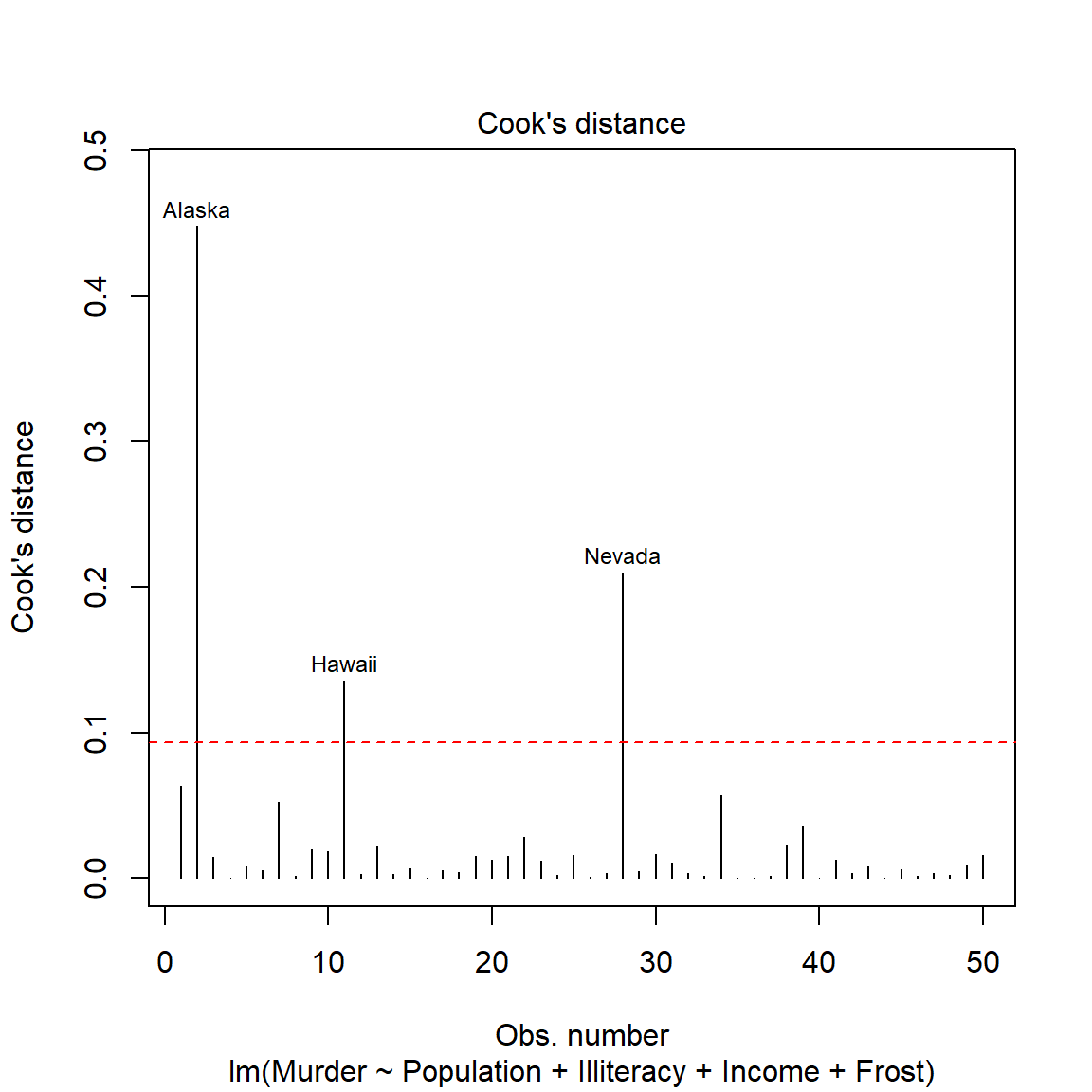Cook’s distance, leverages, and Mahalanobis distance can be effective for finding influential cases when a single outlier exist, but can fail if there are two or more outliers.

Nevertheless, these numerical diagnostics combined with plots such as residuals versus fitted values and fitted values versus the response are probably the most effective techniques for detecting cases that affect the fitted values when the multiple linear regression model is a good approximation for the bulk of the data.

We are going to use the same data example and linear regression model (fit1) for the Cook’s distance plot.

The function influencePlot() from {car} package, is a very handy function to represent these unusual observation issues. This function creates a bubble plot of Studentized residuals versus hat values, with the areas of the circles representing the observations proportional to the value Cook’s distance.

• Vertical reference lines are drawn at twice and three times
• the average hat value, horizontal reference lines at -2, 0, and 2 on the Studentized-residual scale.
• x-axis: Hat-value (with cutoffs)
• Y-axis: studentized residual
• size of bubble (Cook’s D)
# id.n indicates how many most unusual observations are identified.
car::influencePlot(fit1, id.n=3)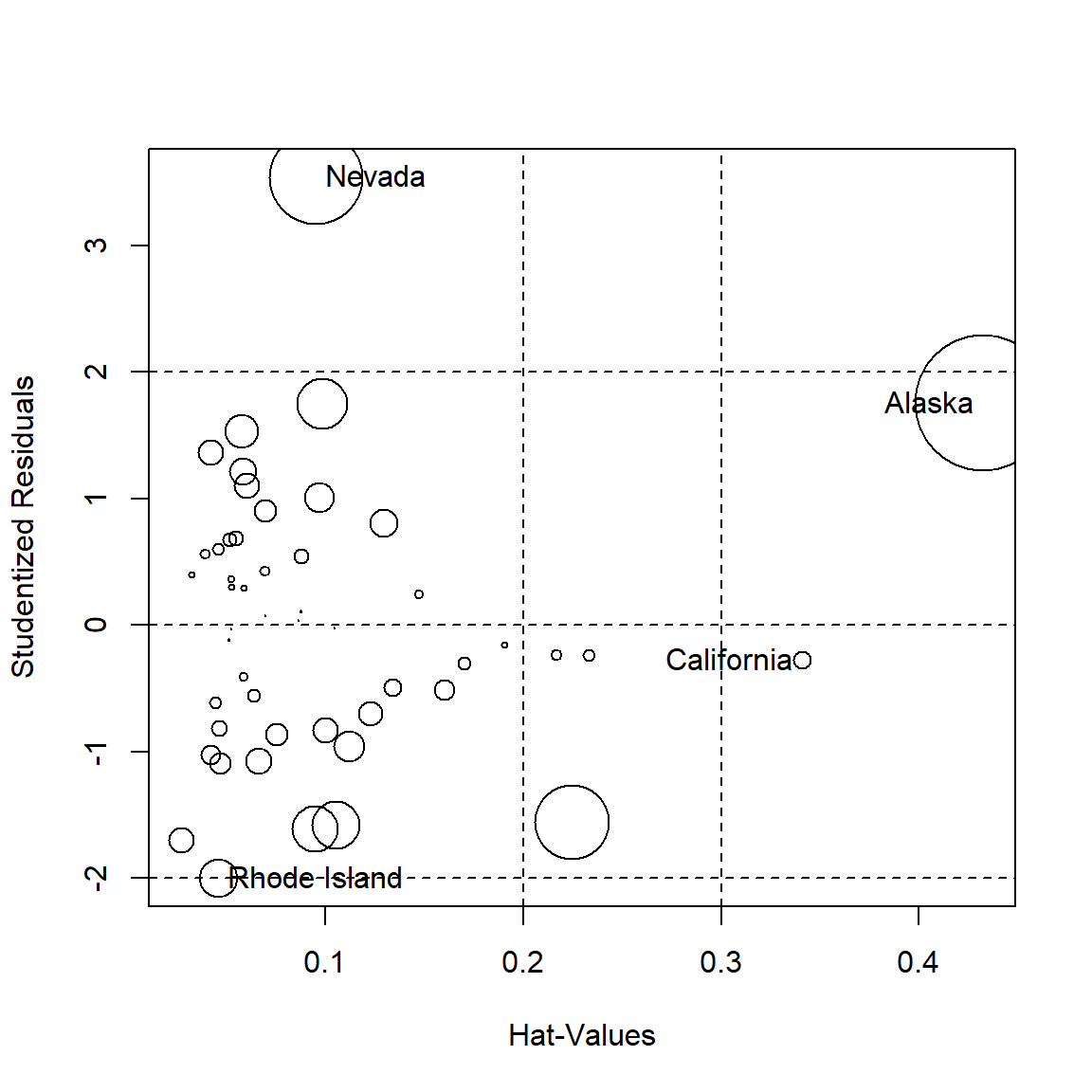##                 StudRes        Hat       CookD
## California   -0.2761492 0.34087628 0.008052956
## Rhode Island -2.0001631 0.04562377 0.035858963

### 2.2.8 DFITS

$$DFITS_{i}$$ is the scaled difference between the predicted responses from the model constructed from all of the data and the predicted responses from the model constructed by setting the $$i_{th}$$ observation aside.

It is similar to Cook’s distance. Unlike Cook’s distance, it does not look at all of the predicted values with the i-th observation set aside.

#### 2.2.8.1 Rule of Thumb

Some analysts suggest investigating observations for which $$|DFITS_{i}|$$ is greater than $$2\sqrt{(p+1)/(n−p−1)}$$. Cook’s D and DFITS give similar answers.

# 3 Summary

• Small samples are especially vulnerable to outliers-there are fewer cases to counter the outlier

• Large samples can also be affected

• Even if you have many cases, and your variables have limited ranges, miscodes that could influence the regression model are still possible

• Unusual cases are only influential when they are both unusual in terms of their Y value given their X (outlier), and when they have an unusual X-value (leverage):

$Influence = Leverage \times Discrepancy$

• We can test for outliers using studentized residuals and quantile-comparison plots

• Leverage is assessed by exploring the hat-values

• Influence is assessed using DFBetas and, preferably Cook’s Ds

• Influence Plots (or bubble plots) are useful because they display the studentized residuals, hat-values and Cook’s distances all on the same plot

• Joint influence is best assessed using Added-Variable Plots (or partial-regression plots)

Note: Some of the contents are originally from Dagmar Blatná’s and William G. Jacoby tutorials. If you are interested in, you can find both online tutorials below.

Dagmar Blatná

William G. Jacoby

– To be Continued##### Ou Zhang
###### Research Scientist & Data Scientist

I’m a research scientist, psychometrician, and data scientist, who loves psychometrics, applied statistics, general data science, and programming.